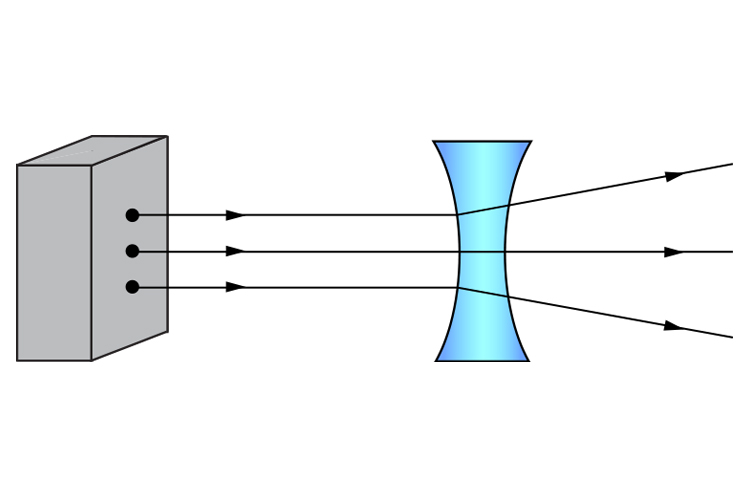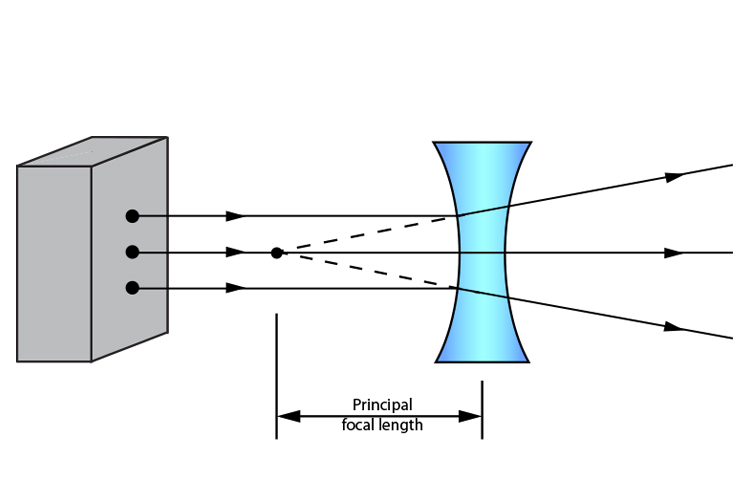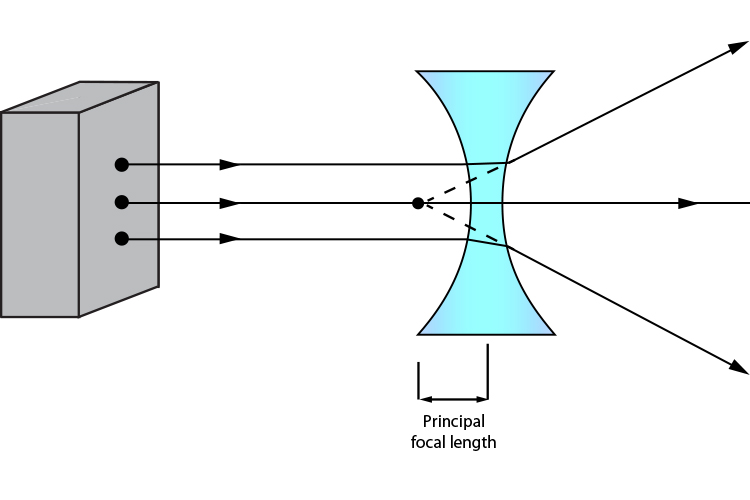# Practical ways to find focal length – concave lens light box

The simplest way to find the principal focal length of a concave lens is to use a light box with three parallel beams.Then project these rays back to where the virtual lines intersect and you will find the principal focal point.Then you can find the principal focal length by measuring between the principal focal point and the centre of the lens.

You will find that if you use a concave lens of greater curvature, the converging rays will be steeper and therefore closer to the lens. The principal focal length will be shorter.